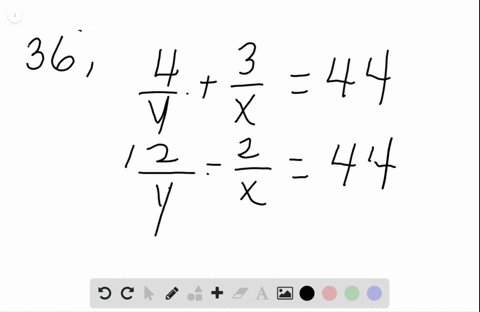🎉 The Study-to-Win Winning Ticket number has been announced! Go to your Tickets dashboard to see if you won! 🎉View Winning Ticket###  \begin{aligned} \text { Find the ratio of } x…

02:23Problem 35

# Points $B$ and $C$ lie on $\overline{A D}$ . Find $A C$ if $\frac{A B}{B D}=\frac{3}{4}, \frac{A C}{C D}=\frac{5}{6},$ and $B D=66$

## Discussion

You must be signed in to discuss.

## Video Transcript

We know that a C over C. D is five divided by sex. Therefore, we know five sex times 63 because remember CDs 63 this gives us 52.5.